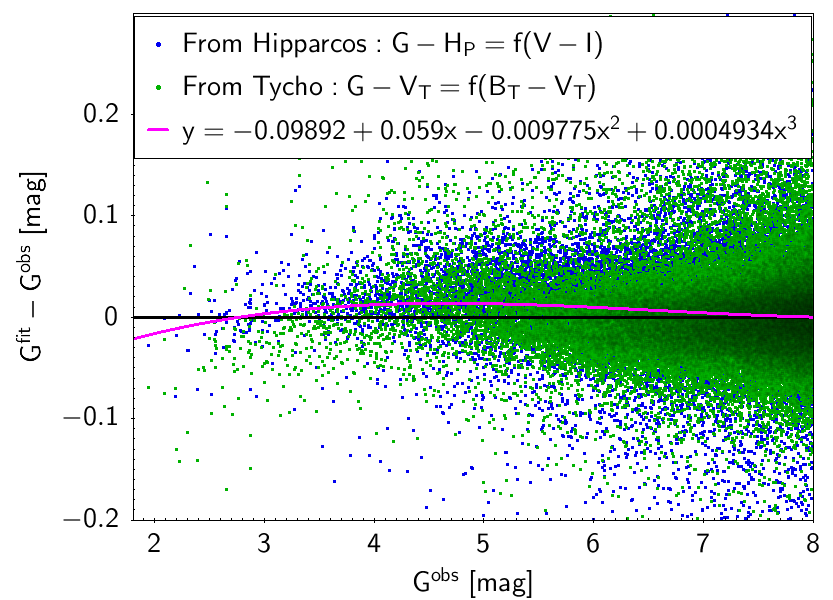5.5.2 Saturation correction

The saturation in the case of bright stars is shown in Figure 5.30. The impact of the saturation on the results of the $G$ fluxes and magnitudes has decreased with respect to Gaia EDR2 because of changes in several steps of the calibrations. The figure shows the residuals when fitting $G-H_{P}$ as a function of $V-I$, or $G-V_{T}$ as a function of $B_{T}-V_{T}$ and plotting them against the magnitude. Tycho-2 and Hipparcos data were combined to derive empirical corrections. The corrected magnitudes from the mean magnitudes in Gaia EDR3, $G^{\rm corr}_{\rm XP}$ can be obtained with the following equations:

 $\displaystyle G^{\rm corr}-G$ $\displaystyle=$ $\displaystyle-0.09892+0.059G-0.009775G^{2}+0.0004934G^{3}$ (5.29) $\displaystyle G_{\rm BP}^{\rm corr}-G_{\rm BP}$ $\displaystyle=$ $\displaystyle-0.9921-0.02598G+0.1833G^{2}-0.02862G^{3}$ (5.30) $\displaystyle G_{\rm RP}^{\rm corr}-G_{\rm RP}$ $\displaystyle=$ $\displaystyle-14.94+14.41G_{\rm RP}-4.657G_{\rm RP}^{2}+0.503G_{\rm RP}^{3}$ (5.31)

The $G$ relationship should only be used in the range $2.0 mag, the $G_{\rm BP}$ relationship only for $2.0 mag, and the $G_{\rm RP}$ relationship only for $2.0 mag.Figure 5.30: Saturation correction at the bright end for G (top), GBP (center) and GRP (bottom) passbands.